# Quality of Sewage Notes | Study Environmental Engineering - Civil Engineering (CE)

## Civil Engineering (CE): Quality of Sewage Notes | Study Environmental Engineering - Civil Engineering (CE)

The document Quality of Sewage Notes | Study Environmental Engineering - Civil Engineering (CE) is a part of the Civil Engineering (CE) Course Environmental Engineering.
All you need of Civil Engineering (CE) at this link: Civil Engineering (CE)

Aerobic Decomposition

1. Nitrogenous organic matter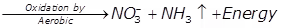Nitrate
2. Carbonaceous organic matter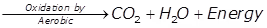Carbon dioxide
3. Sulphurous organic matter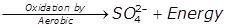Sulphate
Nitrogen Cycle under Aerobic Decomposition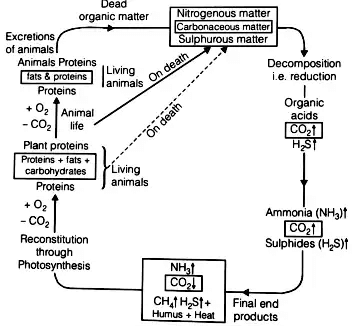Anaerobic Decomposition
1. Nitrogenous organic matter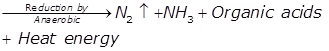2. Carbonaceous organic matter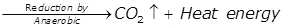3. Sulphurous organic matter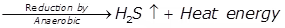4. Organic acids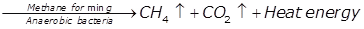Threshold Odour Number (TON)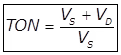Vs = The volume of the sewage
VD = The volume of distilled water or odourless water.

Total Solids, Suspended Solids and Settleable Solids
1.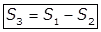Where,
S3 = Dissolved solids plus colloidal or filterable solids in mg/lit
S2 = Non-filterable solids in mg/lit
S1 = The total amount of solids in mg/lit
S4 = Volatile suspended solids, in (mg/lit.)
S5 = Fixed solids
2.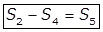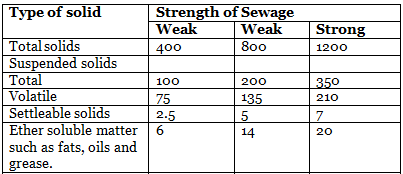Total Solids
50% → Dissolved
25% → Suspended
25% → Settleable

Chemical Oxygen Demand

2. K2Cr2O7 + H2SO4 added and used is measured.

Theoretical Oxygen Demand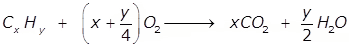C6H6 + 7.5O2 → 6O2 + 3H2O
Benzene
C6H12O6 + 6O2 → 6O+ 6H2O
Glucose

Biochemical Oxygen Demand
1.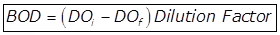Where,
Biochemical oxygen demand in ppm or mg/lit.
Initial dissolved oxygen in mg/lit.
Final dissolved oxygen in mg/lit.

2.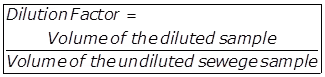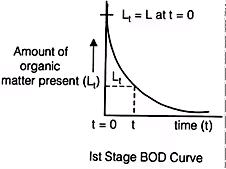(i)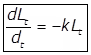Where,
k = Rate constant signifying the rate of oxidation of organic matter and it depends upon the nature of organic matter and temperature. Its unit is per day.
Lt = O2 the equivalent of organic matter present after t days.
(ii)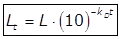Where,
kD = Deoxygenation constant.
L = Organic matter present at t = 0
(iii)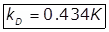(iv)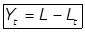Where,
Yt = The total amount of organic matter oxidized int days i.e. BOD.
(v)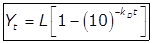(vi)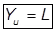Where,
Yu = Ultimate B.O.D of  days.
(vii)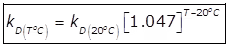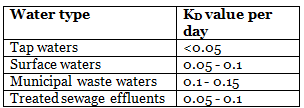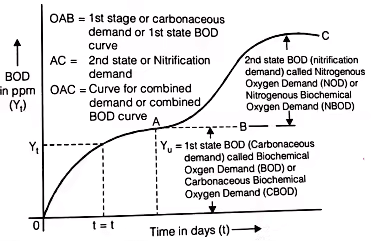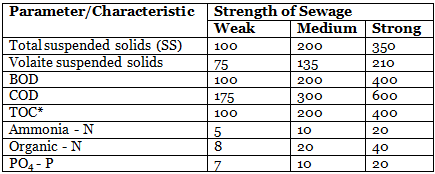(viii) Laboratory Estimations of kD and L values
(Thomas Method)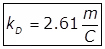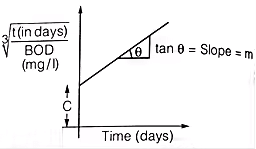Where,
m = Slope of the line
C = Intercept of the line on the y-axis.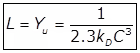Relative Stability (s)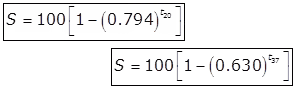Where
t20 = time in days at 20oC.
t37 = time in days at 37oC.

The document Quality of Sewage Notes | Study Environmental Engineering - Civil Engineering (CE) is a part of the Civil Engineering (CE) Course Environmental Engineering.
All you need of Civil Engineering (CE) at this link: Civil Engineering (CE)Use Code STAYHOME200 and get INR 200 additional OFF

## Environmental Engineering

14 videos|74 docs|70 tests

Track your progress, build streaks, highlight & save important lessons and more!

,

,

,

,

,

,

,

,

,

,

,

,

,

,

,

,

,

,

,

,

,

;